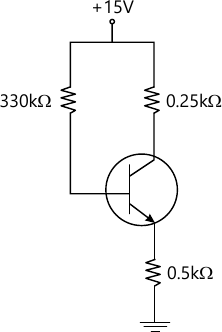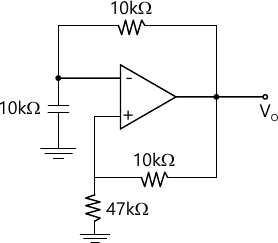MORE IN Analog and Digital Electronics
VTU Computer Science (Semester 3)
Analog and Digital Electronics
December 2015
Total marks: --
Total time: --
INSTRUCTIONS
(1) Assume appropriate data and state your reasons
(2) Marks are given to the right of every question
(3) Draw neat diagrams wherever necessary

1 (a) What is an operating point? How to choose an operating point for faithful amplification of an input signal?
6 M
1 (b) Derive the expressions for the operation point in voltage divider bias configuration. Use accurate method for analysis.
8 M
1 (c) For the circuit shown in Fig Q1(c), calculate IB, IC, VCE, VC, VB and VE Assume β=100 and VBE=0.7V6 M

2 (a) Bring out the difference between Bipolar Junction Transistors and Field effect Transistors.
5 M
2 (b) Explain the construction and working of N-channel depletion mode MOSFET along with its characteristics curves.
10 M
2 (c) List and briefly explain some applications of field effect transistors.
5 M

3 (a) Define the following terms with reference to photo sensors
i) Responsivity
ii) Response time
iii) Noise equivalent power
iv) Spectral Response.
7 M
3 (b) Explain the working of a photo diode along with its VI characteristics.
7 M
3 (c) Write a short note on Liquid crystal displays.
5 M

4 (a) With a neat diagram, explain the h-parameter model for common-emitter transistor configuration.
8 M
4 (b) Explain bandwidth with reference to an amplifier. What are the factors affecting it?
5 M
4 (c) Explain the importance of cascaded connection of amplifiers, with a diagram.
7 M

5 (a) Classify large signal amplifier and make a suitable comparison.
10 M
5 (b) With a block diagram explain the working no Negative feedback amplifiers. How is gain affected in these amplifiers?
10 M

6 (a) Explain Barkhausen criterion.
6 M
6 (b) Determine the gain and phase shift for an oscillator circuit with a 1% positive feedback and a two stage CE configuration.
4 M
6 (c) Explain the working of an Astable Multivibrator with necessary diagrams and expression for frequency of oscillations.
10 M

7 (a) What is voltage Regulation? With a neat circuit diagram explain the working of a Buck regulator.
12 M
7 (b) Compare linear power supplies with switched mode power supplies.
3 M
7 (c) A regulated power supply provides a ripple rejection of -80dB. If the ripple voltage in an unregulated input were 2V, determine the output ripple.
5 M

8 (a) Discuss any five performance parameters of an operational Amplifier.
5 M
8 (b) Explain with neat diagrams, the working of low-pass and high pass filters using operational amplifiers.
8 M
8 (c) For the relaxation oscillator circuit in Fig. Q8(c), determine the peak ? to ? peak amplitude and frequency of the square wave given that saturation output voltage of op-amp is ± 12.5V at power supply voltages of ± 15V.7 M

More question papers from Analog and Digital Electronics